## Introduction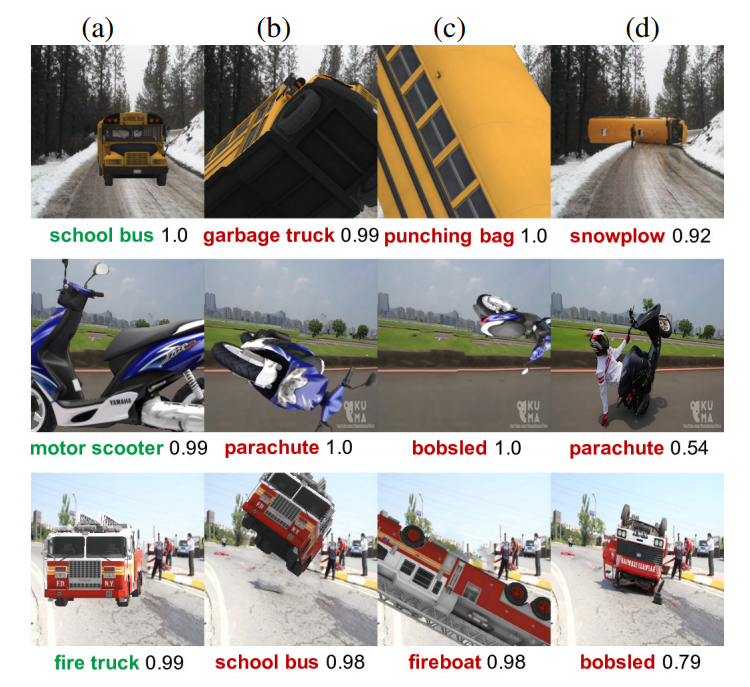While convolutional neural networks (CNNs) have become increasingly good at object classification, adversarial attacks have come to challenge this expertise. While most adversarial attack research has been done by modifying pixels to fool classifiers, the authors suggest that CNNs can be fooled by Out of Distribution (OoD) poses of otherwise easily classified objects.

## Out of Distribution poses

To formalize the problem of finding poses, the authors try to optimize the following problem: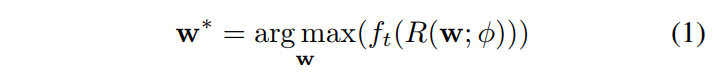With $$w$$ being the set of parameters for a 3D rotation and translation, $$f$$ being the classifier, $$t$$ the incorrect class, $$R$$ the rendered image and $$\phi$$ the image parameters such as lighting, background image, vertices, texture, etc. They calculated the partial derivatives of $$w$$ and performed vanilla gradient-descent to minimize the cross-entropy loss $$L$$ w.r.t. a certain class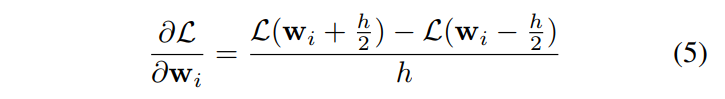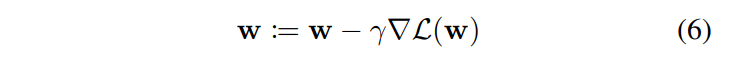To backpropagate gradients to scene parameters, they both used a differentiable 3D renderer (github) and calculated gradient analytically, which was possible due to their small number of parameters (6). They also used random search (RS) to find parameters.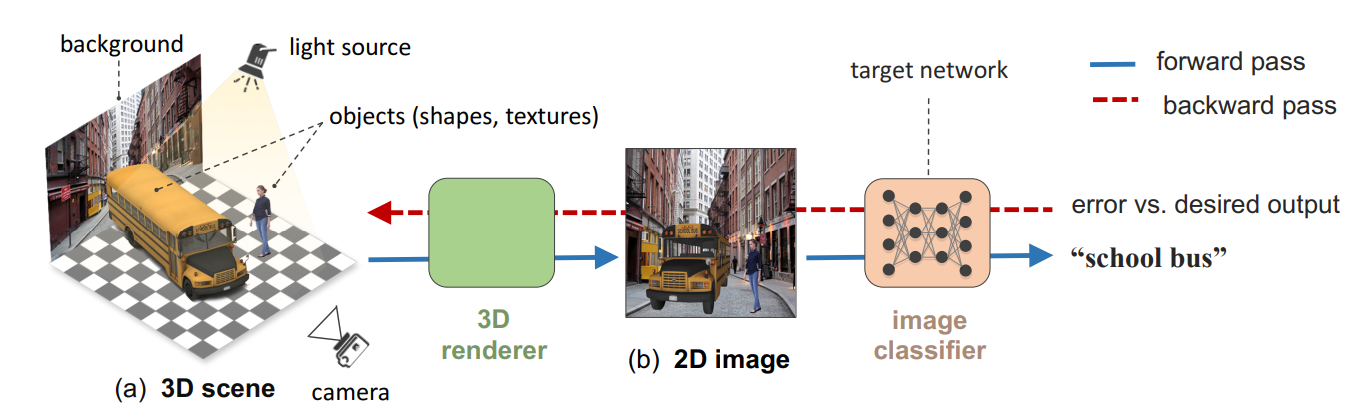## Results

The authors first trained on Inception-v3 and ImageNet and found that the model was highly sensitive to pitch and roll perturbations.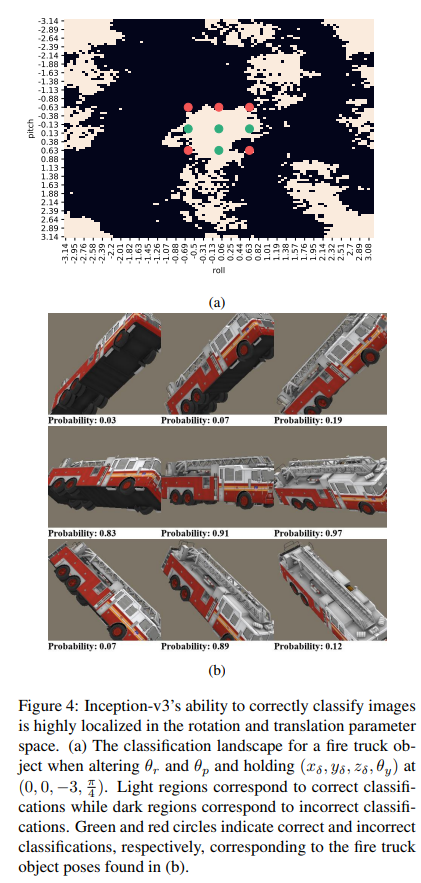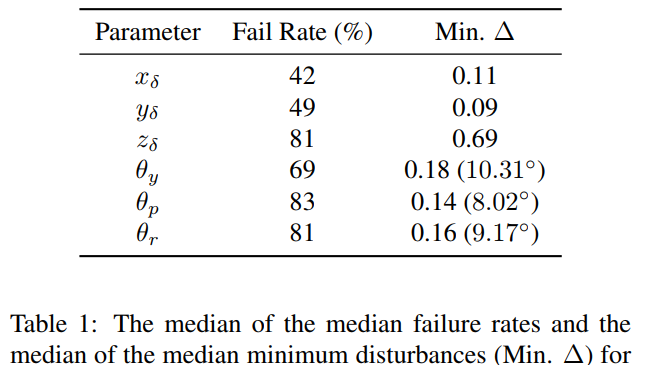They also found that misclassification rates transferred highly to AlexNet (99.9%) and Resnet-50(99.4%), both trained on ImageNet and tested on the same adversarial examples. They also found that 75.5% of adversarial poses generated for Inception-v3 caused misclassification errors of the object detector YOLOv3 trained on MS-COCO. Finally, they tried Adversarial Training on AlexNet and got encouraging results.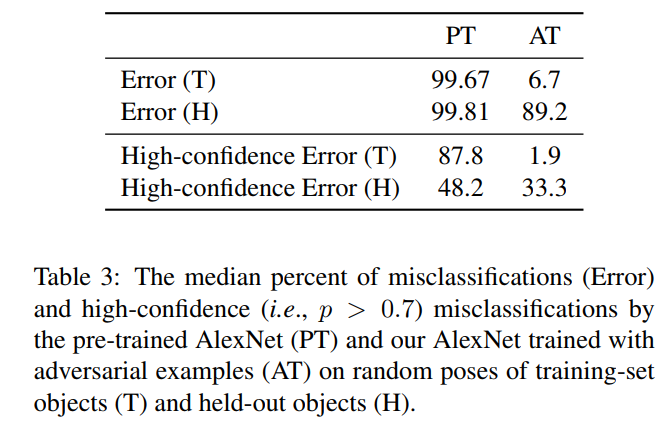Github link of project : https://github.com/airalcorn2/strike-with-a-pose